Electron. J. Diff. Equ., Vol. 2015 (2015), No. 153, pp. 1-13.

### Inverse coefficient problem for the semi-linear fractional telegraph equation Halyna Lopushanska, Vitalia Rapita

Abstract:
We establish the unique solvability for an inverse problem for semi-linear fractional telegraph equationwith regularized fractional derivatives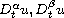of orders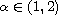,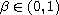with respect to time on bounded cylindrical domain. This problem consists in the determination of a pair of functions: a classical solutionof the first boundary-value problem for such equation, and an unknown continuous coefficient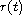under the over-determination conditionwith given functions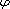and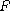.

Submitted April 22, 2015. Published June 11, 2015.
Math Subject Classifications: 35S15.
Key Words: Fractional derivative; inverse boundary value problem; over-determination integral condition; Green's function; integral equation.

Show me the PDF file (261 KB), TEX file, and other files for this article.Halyna Lopushanska Department of Differential Equations Ivan Franko National University of Lviv Lviv, Ukraine email: lhp@ukr.net Vitalia Rapita Department of Differential Equations Ivan Franko National University of Lviv Lviv, Ukraine email: vrapita@gmail.com